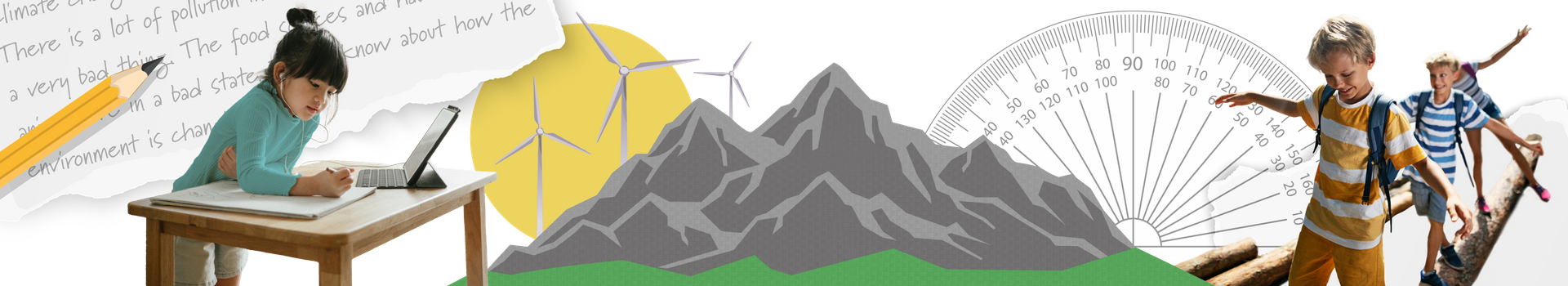In Grade 4 Mathematics, our students embark on their path to junior math mastery by building on their number, fraction, and decimal skills and applying the four operations with larger numbers in their local community, around the world, and around the solar system. They will also learn to measure and notice patterns and geometric attributes in the real world such as in crafts and at construction sites. Their financial literacy will also explore budgets and spending decisions. Through coding, they will discover loops within loops as they control a drawing program to create shapes. This course is designed to be 200-300 instructional hours.

Curriculum Information: Mathematics (2020)

Developed by: D2L

Development Date: 2020

Please note that elementary courses do not have prerequisites, and Ontario schools do not issue transcript credits at the elementary level. All courses are available in the facilitated and independent pathways.

Facilitated

Independent

#### Course Outline

Representing Numbers

In the Representing Numbers unit, students learn to read and write numbers up to 10 000, show an understanding of place value up to 10 000, represent, compare, and order numbers up to 10 000. They also round four-digit numbers in real-life situations.

Geometry

In the Geometry unit, students draw lines of symmetry on 2D shapes, and identify, compare, and classify quadrilaterals. They learn how to identify various angles. Students identify, perform, and describe reflections and learn to create patterns using reflections.

In the Addition and Subtraction unit, students add and subtract four-digit numbers using partial sums, add and subtract four-digit numbers vertically,

Multiplication and Division

In the Multiplication and Division unit, students multiply ad multiply and divide numbers from 1 to 10. They multiply and divide by 10, 100, and 1 000 using mental strategies and multiply and divide using a variety of tools. Students solve vertical multiplication equations, divide two-digit and three-digit by one-digit numbers. Students describe relationships using multiplication, and explore multiplication and unit rates. They also solve problems involving more than one operation.

Patterning and Algebra

In the Patterning and Algebra unit, students create and extend patterns, analyze terms and term numbers, create number patterns, and predict terms in a pattern. Students also determine the relationship between multiplication and division, find the missing number in a multiplication problem, and identify the commutative and distributive properties.

Fractions and Decimal Numbers

In the Fractions and Decimal Numbers unit, students represent fractions, identify the fraction of a group, compare and order fractions, show equivalent fractions, and count by halves, thirds, fourths, and tenths. Students also read and write decimal numbers, show place values from 0.1 to 10 000, represent, compare, and order decimal numbers up to tenths, count forward, add and subtract decimal numbers, and explore the relationship between fractions and decimal numbers.

Coding

In the Coding unit, students learn how to write and execute code to draw shapes. They learn the concepts of variables and nested events. Students learn how to debug code with nested events.

Data

In the Data unit, students collect data by conducting a survey and collect and organize data in charts, tables, and graphs. They learn how to create an infographic to represent data. Students read, interpret, and draw conclusions on data, demonstrate an understanding of the median, describe and compare sets of data, and explore probability and probability experiments.

Measurement

In the Measurement unit, students estimate, measure, and record mass. Students investigate grams and kilograms, capacity, millilitres and litres, and volume. They also compare mass and capacity. Students determine elapsed time and solve problems involving years, decades, and centuries.

Financial Literacy

In the Financial Literacy unit students identify different methods of payment and learn how to estimate and calculate cost using addition. They calculate the change for a cash purchase. Students explore spending, saving, earning, investing, and donating while exploring the concept of good purchases.

Socio-Emotional Learning

Throughout the course, students will be encouraged to build their social emotional learning skills by focusing specifically on the interactions and challenges they face in the world around them. They will learn to solve problems using reasoning, cooperation, tools, and a variety of different problem-solving strategies. Students will practice using math language in order to communicate their ideas and solutions in order to tell a story and to cooperate with others.

#### Required Resources

This course is entirely online and does not require nor rely on any textbook. Students will require the following resources:

• A scanner, smartphone camera, or similar device to digitize handwritten or hand-drawn work
• A device to record audio
• A printer
• A physical binder, folder, or notebook for offline activities
• Scissors, stapler, ruler, glue, baking soda, salt, dish soap, food colouring, vinegar, cardboard, dice, a plastic bottle
• Various household items to complete offline activities

#### Teaching and Learning Strategies

Through a balance of problem-solving and direct instruction, students develop a strong foundation of mathematical processes, knowledge, and skills to apply in real-world contexts. The course engages multiple learning styles by combining technology and offline activities that provide opportunities to develop an understanding of skills and concepts in interactive and concrete ways. The lessons feature a variety of intriguing storylines, videos, graphics, and interactive games to reinforce students’ learning. The activities also build a foundation of mathematical models and strategies that students will use throughout their elementary grades.

The course relies on the assistance of a learning coach who supports young students as they move through the content. The learning coach will be involved in facilitating technical aspects of the course and in participating in discussion-based activities to assist students in developing communication skills.